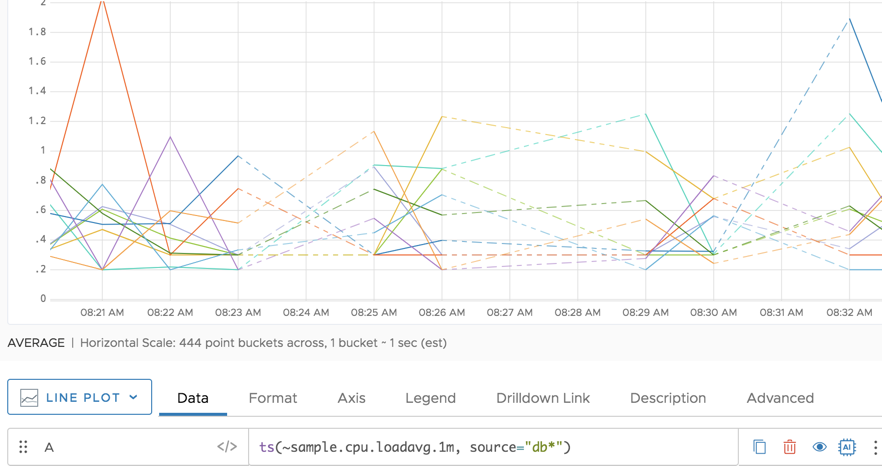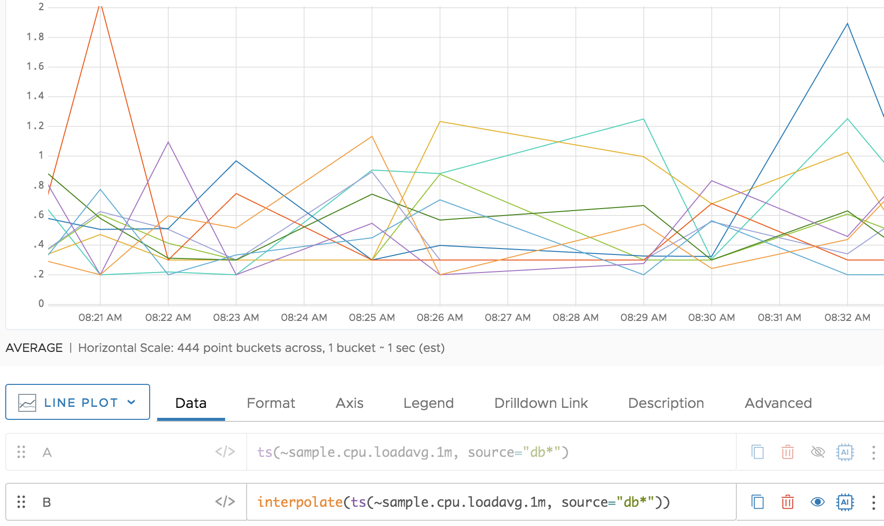Reference to the interpolate() function

## Summary

``````interpolate(<tsExpression>)
``````

Fills in gaps in each time series described by `tsExpression`, by inserting a continuous linear interpolation of data points.

## Parameters

ParameterDescription
tsExpression Expression that describes the time series in which to replace data gaps with interpolated data points.

## Description

The `interpolate()` function replaces a gap of missing data (dashed line) with a solid line. The value assigned to the new solid line depends on where the interpolation occurred in relation to the value on the y-axis.

For example, suppose there is a gap of missing data in between two reported point values of 2 and 1. The value assigned to that gap of missing data will be between 2 and 1, with the displayed value dependent on where it intersects the y-axis. When the query engine performs interpolation, it applies `interpolate()` to the first 85% of a chart window.

## Examples

The following example zooms in on an area of a chart where data are missing. In charts, you’ll see dashed lines to indicate the missing data.When you use `interpolate()` for this use case, the dashed lines are replaced with filled lines, indistinguishable from areas where data are available.